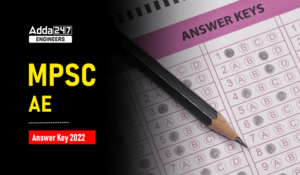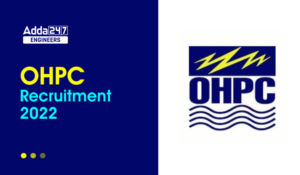Engineering Jobs   »   DMRC-JE BASED QUIZ CIVIL ENGINEERING (SOIL...

# DMRC-JE BASED QUIZ CIVIL ENGINEERING (SOIL MECHANICS)

Attempt civil engineering quiz for DMRC-JE. This quiz contains 10 questions of Soil Mechanics with their relevant solutions.

Q1. Which of the following is not an assumption in Terzaghi’s one-dimensional consolidation theory?

(a) Darcy’s Law is applicable

(b) Soil is Laterally confined

(c) Soil is hetrogeneous

(d) Soil is fully saturated

Q2. Sand particles are made of

(a) Kaolinite

(b) Illite

(c) Montmorrilonite

(d) Quartz

Q3. A soil has a bulk density of 22 kN/m³ and water content 10% the dry density of soil is ?

(a) 18.6 kN/m³

(b) 20 kN/m³

(c) 22 kN/m³

(d) 23.2 kN/m³

Q4. Density Index of soil express its :

(a) Hardness

(b) Friction

(c) Smoothness

(d) Relative compactness

Q5. Which one in the following list does not posses plasticity?

(a) Bentonite

(b) Kaolinite

(c) Rock Flour

(d) Fat clay

Q6. If permeability of a soil at the void ratio of e₁, e₂ and k₁, k₂ then which of the following relation is correct?

(a) k_1/k_2 = (1+e_1)/(1+e_2 )
(b) k_1/k_2 = (1–e_1)/(1–e_2 )
(c) k_1/k_2 = e_1/e_2
(d) None of the above

Q7. Which of the following roller types is the most suitable for compacting fatty clay in field?

(a) Sheepfoot

(b) Rubber tyred

(c) Tandem

(d) Vibratory

Q8. When consolidation of saturated soil sample occurs, the degree of saturation.

(a) Increase

(b) decrease

(c) remain constant

(d) may increase or decrease

Q9. Coulomb’s equation for shear strength can be represented by :

(a) C = S + σ_n tan ϕ

(b) S = C – σ_n tan ϕ

(c) C = σ_n+ S tan ϕ

(d) S = C + σ_n tan ϕ

Q10. Drainage conditions are best controlled during :

(a) Direct shear box text

(b) Vane shear test

(c) Unconfined compression test

(d) Triaxial test

Solutions

S1. Ans.(c)

S2. Ans.(d)

S3. Ans.(b)
Sol. γ_b = 22 kN/m³
ω = 10%
γ_d = γ_b/(1+ω) = 22/(1+0.1)
⇒ 22/1.1
⇒ 20 kN/m³

S4. Ans.(d)

S5. Ans.(c)

S6. Ans.(d)

Sol. kαe²

k_1/k_2 =(e_1/e_2 )^2

S7. Ans.(a)

S8. Ans.(c)

S9. Ans.(d)

Sol. S = C +σ_n  tan ϕ

S = shear strength

ϕ = total frictional Angle

C = Unit cohesion

σ_n= Normal stress

S10. Ans.(d)

Sharing is caring!

Thank You, Your details have been submitted we will get back to you.
•BEL Recruitment 2022 Notification PDF Ou...
•Jaipur Development Authority Recruitment...
•MPSC AE 2022 Answer Key Out, Download MP...
•AAI Assistant Recruitment 2022, Junior a...
•OHPC Recruitment 2022 Notification PDF O...
•Ordnance Factory Medak Recruitment 2022 ...